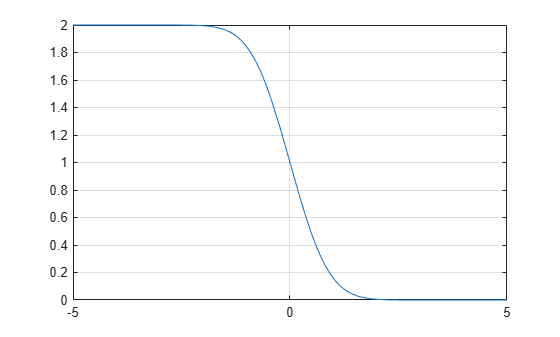# erfc

Complementary error function

## Syntax

``erfc(X)``
``erfc(K,X)``

## Description

example

````erfc(X)` represents the complementary error function of `X`, that is,```erfc(X) = 1 - erf(X)```.```

example

````erfc(K,X)` represents the iterated integral of the complementary error function of `X`, that is, `erfc(K, X) = int(erfc(K - 1, y), y, X, inf)`.```

## Examples

### Complementary Error Function for Floating-Point and Symbolic Numbers

Depending on its arguments, `erfc` can return floating-point or exact symbolic results.

Compute the complementary error function for these numbers. Because these numbers are not symbolic objects, you get the floating-point results:

`A = [erfc(1/2), erfc(1.41), erfc(sqrt(2))]`
```A = 0.4795 0.0461 0.0455```

Compute the complementary error function for the same numbers converted to symbolic objects. For most symbolic (exact) numbers, `erfc` returns unresolved symbolic calls:

`symA = [erfc(sym(1/2)), erfc(sym(1.41)), erfc(sqrt(sym(2)))]`
```symA = [ erfc(1/2), erfc(141/100), erfc(2^(1/2))]```

Use `vpa` to approximate symbolic results with the required number of digits:

```d = digits(10); vpa(symA) digits(d)```
```ans = [ 0.4795001222, 0.04614756064, 0.0455002639]```

### Error Function for Variables and Expressions

For most symbolic variables and expressions, `erfc` returns unresolved symbolic calls.

Compute the complementary error function for `x` and ```sin(x) + x*exp(x)```:

```syms x f = sin(x) + x*exp(x); erfc(x) erfc(f)```
```ans = erfc(x) ans = erfc(sin(x) + x*exp(x))```

### Complementary Error Function for Vectors and Matrices

If the input argument is a vector or a matrix, `erfc` returns the complementary error function for each element of that vector or matrix.

Compute the complementary error function for elements of matrix `M` and vector `V`:

```M = sym([0 inf; 1/3 -inf]); V = sym([1; -i*inf]); erfc(M) erfc(V)```
```ans = [ 1, 0] [ erfc(1/3), 2] ans = erfc(1) 1 + Inf*1i```

Compute the iterated integral of the complementary error function for the elements of `V` and `M`, and the integer `-1`:

```erfc(-1, M) erfc(-1, V)```
```ans = [ 2/pi^(1/2), 0] [ (2*exp(-1/9))/pi^(1/2), 0] ans = (2*exp(-1))/pi^(1/2) Inf```

### Special Values of Complementary Error Function

`erfc` returns special values for particular parameters.

Compute the complementary error function for x = 0, x = ∞, and x = –∞. The complementary error function has special values for these parameters:

`[erfc(0), erfc(Inf), erfc(-Inf)]`
```ans = 1 0 2```

Compute the complementary error function for complex infinities. Use `sym` to convert complex infinities to symbolic objects:

`[erfc(sym(i*Inf)), erfc(sym(-i*Inf))]`
```ans = [ 1 - Inf*1i, 1 + Inf*1i]```

### Handling Expressions That Contain Complementary Error Function

Many functions, such as `diff` and `int`, can handle expressions containing `erfc`.

Compute the first and second derivatives of the complementary error function:

```syms x diff(erfc(x), x) diff(erfc(x), x, 2)```
```ans = -(2*exp(-x^2))/pi^(1/2) ans = (4*x*exp(-x^2))/pi^(1/2)```

Compute the integrals of these expressions:

```syms x int(erfc(-1, x), x)```
```ans = erf(x)```
`int(erfc(x), x)`
```ans = x*erfc(x) - exp(-x^2)/pi^(1/2)```
`int(erfc(2, x), x)`
```ans = (x^3*erfc(x))/6 - exp(-x^2)/(6*pi^(1/2)) +... (x*erfc(x))/4 - (x^2*exp(-x^2))/(6*pi^(1/2))```

### Plot Complementary Error Function

Plot the complementary error function on the interval from -5 to 5.

```syms x fplot(erfc(x),[-5 5]) grid on```## Input Arguments

collapse all

Input, specified as a symbolic number, variable, expression, or function, or as a vector or matrix of symbolic numbers, variables, expressions, or functions.

Input representing an integer larger than `-2`, specified as a number, symbolic number, variable, expression, or function. This arguments can also be a vector or matrix of numbers, symbolic numbers, variables, expressions, or functions.

collapse all

### Complementary Error Function

The following integral defines the complementary error function:

`$erfc\left(x\right)=\frac{2}{\sqrt{\pi }}\underset{x}{\overset{\infty }{\int }}{e}^{-{t}^{2}}dt=1-erf\left(x\right)$`

Here `erf(x)` is the error function.

### Iterated Integral of Complementary Error Function

The following integral is the iterated integral of the complementary error function:

`$erfc\left(k,x\right)=\underset{x}{\overset{\infty }{\int }}erfc\left(k-1,y\right)dy$`

Here, $erfc\left(0,x\right)=erfc\left(x\right)$.

## Tips

• Calling `erfc` for a number that is not a symbolic object invokes the MATLAB® `erfc` function. This function accepts real arguments only. If you want to compute the complementary error function for a complex number, use `sym` to convert that number to a symbolic object, and then call `erfc` for that symbolic object.

• For most symbolic (exact) numbers, `erfc` returns unresolved symbolic calls. You can approximate such results with floating-point numbers using `vpa`.

• At least one input argument must be a scalar or both arguments must be vectors or matrices of the same size. If one input argument is a scalar and the other one is a vector or a matrix, then `erfc` expands the scalar into a vector or matrix of the same size as the other argument with all elements equal to that scalar.

## Algorithms

The toolbox can simplify expressions that contain error functions and their inverses. For real values `x`, the toolbox applies these simplification rules:

• ```erfinv(erf(x)) = erfinv(1 - erfc(x)) = erfcinv(1 - erf(x)) = erfcinv(erfc(x)) = x```

• ```erfinv(-erf(x)) = erfinv(erfc(x) - 1) = erfcinv(1 + erf(x)) = erfcinv(2 - erfc(x)) = -x```

For any value `x`, the system applies these simplification rules:

• `erfcinv(x) = erfinv(1 - x)`

• `erfinv(-x) = -erfinv(x)`

• `erfcinv(2 - x) = -erfcinv(x)`

• `erf(erfinv(x)) = erfc(erfcinv(x)) = x`

• `erf(erfcinv(x)) = erfc(erfinv(x)) = 1 - x`

 Gautschi, W. “Error Function and Fresnel Integrals.” Handbook of Mathematical Functions with Formulas, Graphs, and Mathematical Tables. (M. Abramowitz and I. A. Stegun, eds.). New York: Dover, 1972.